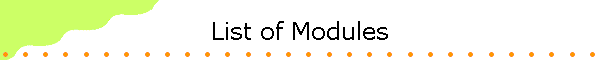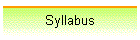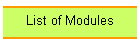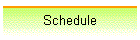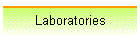• Module 1:  Waves and Light
Mechanical waves, electromagnetic waves, photons, QED
• Mechanical waves
• Electromagnetic waves
• Photons
• Module 2:  Quantum Mechanics
Quantum Mechanics and probability, the uncertainty principle, the Schroedinger equation
• Matter waves and the uncertainty principle
• Wave equations
• The Schroedinger equation
• One-dimensional problems
• Three-dimensional problems
• Module 3:  Atoms
Quantization of energy and angular momentum, the exclusion principle, atomic structure
• The hydrogen atom
• Electron spin
• Multi-electron atoms
• Module 4:  Quantum effects in large systems
Lasers, semiconductors, diodes and transistors, superconductors and superfluids
• Lasers
• Quantum effects in large systems
• Semiconductors
• Module 5  Nuclear Physics
The nucleus, radioactive decay, fission and fusion
• Properties of nuclei
• Nuclear decay
• Nuclear models
• Nuclear energy
• Module 6:  Particles
Leptons and Quarks, the basic interactions
• Particle classification
• The standard model